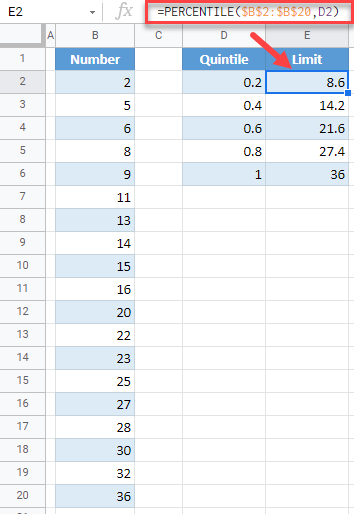# Calculate Quintiles – Excel & Google Sheets

This tutorial demonstrates how to calculate quintiles in Excel and Google Sheets.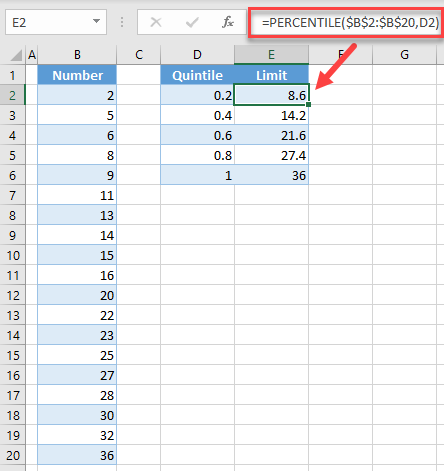## What are Quintiles?

Quintiles separate a dataset into five equal parts. The quintiles return the values at the following percentiles: 20%, 40%, 60%, 80%, and 100%.

## Calculate Quintiles in Excel

We will calculate quintiles for the following dataset: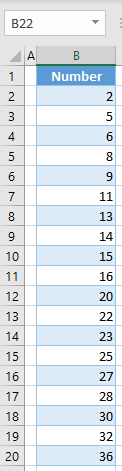In order to achieve this, you have to use the PERCENTILE Function. Follow the next steps.

1. In the helper column (D), enter percentage limits in decimal format.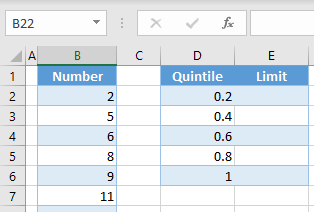2. In cell E2, enter the formula:

``=PERCENTILE(\$B\$2:\$B\$20,D2)``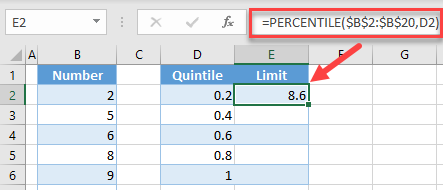Note: Be sure to lock dataset cell references with \$ so that you can copy + paste the formula down the column.

3. Copy the formula down to row 6 in order to get limits for all quintiles.As a result, in column E, you have limits for each quintile. This means that 20% of numbers in the dataset are smaller than 8.6, 40% are smaller than 14.2, etc.

## Calculate Quintiles in Google Sheets

Similar to Excel, you can also calculate quintiles in Google Sheets. To do this, enter the next formula in cell E2, and copy it down to row 6:

``````=PERCENTILE(\$B\$2:\$B\$20,D2)
``````